Published: 30 June 2016

# Numerical optimization of vehicle noises in multi-peak frequency points based on hybrid genetic algorithm and simulated annealing

Xiao-jun Qu1
Hai-long Liang2
Bo-chao Zhang3
Xu-yang Cui4
1, 2, 3North University of China (Shuozhou), Shuozhou, China
4School of Mechanical Engineering, Tianjin University, Tianjin, China
Corresponding Author:
Bo-chao Zhang
Views 65

#### Abstract

The finite element model of Body in White was built, and the corresponding modes were computed in this paper. These computational modes were then compared with experimental results. The small errors showed that the accuracy of the finite element model can satisfy the computational requirements. Based on the verified finite element model, acoustic cavities in the vehicle were extracted to build a boundary element model. Sound pressure levels at all passengers in the vehicle were then computed, compared and analyzed. Results indicated that the sound pressure curve had 6 peak noises. Using the characteristic frequency weight coefficient and field point weight coefficient, the body panels which made large acoustic contributions to the comprehensive sound field under multi-characteristic frequencies were determined. Finally, the improved genetic algorithm based on simulated annealing was used to optimize the key body panels, and peak noises at researched field points after the optimization were further computed. The computational results were compared with those of the original structure, which presented that the noise was improved at most frequency points in the spectrum and peak noises were suppressed obviously.

## 1. Introduction

With the rapid development of light-weight technologies, the dynamic performance of the vehicle has been improved obviously. However, vibrations and noises in the low frequency have also been increased . Loudness of noises in the low frequency was not very large, but long-term noises can very easily cause discomfort and driving safety . Currently, researches on noises in the low frequency have obtained a lot of achievements. Citarella  applied the boundary element method to research acoustic responses and panel contributions, and found the panels which had large influences on driver’s noises. However, the research was not verified by the corresponding experiment, and measures were not adopted to improve noises. Sung  applied the finite element method to analyze noises in the vehicle and considered the coupling between body structures and sound field, finding that the coupling had serious influences on sound field. Langley  applied the boundary element method to predict sound radiation in the structure, but the adopted vibration excitation was only the unit harmonic force and did not satisfy actual situations. Chen  researched the instant values of sound pressures in 20 Hz~250 Hz based on the same road surface and different speeds, but effective values of sound pressures in the analyzed frequency band were not computed. Ding  applied the dynamic substructure method based on vibro-acoustic coupling to research the quantitative relations between noises and parameters of a suspension. Mohanty  applied the finite element method and the boundary element method to analyze the sound field of a truck, and computed the structural vibration response, finding that the noise in the vehicle was improved by adding sound absorption materials.

However, the mentioned researches mainly focused on panel contributions to a single response peak. As for a characteristic field point with multiple peak noises, the panel always had different contributions and characteristics to the different peaks, so that its improvement can hardly be determined. Meanwhile, a single field point cannot completely reflect acoustic characteristics of the full sound field, while the improvement of noises at one field point may cause deterioration of noises at other field points. Han [9, 10] proposed that the weighted summarization could be used to compute panel contributions to full acoustic characteristics in the vehicle and obtained outstanding noise reduction effects using the damping technology, but he had not adopted any optimization algorithm to conduct on an optimization analysis. Panel contributions based on acoustic transfer vector method were conducted in this paper. Then, the characteristic frequency weight coefficient and field point weight coefficient which can be used to determine the key panels were proposed. Finally, the improved genetic algorithm was used to optimize the sound field.

## 2. Theories of acoustic transfer vector

As the natural characteristics of a system, the acoustic transfer vector was a linear relation between the vibration velocity in the structural surface and sound pressures. When the pressure disturbance was very small, the acoustic equation can be considered as linear. A linear relation can be built between the input and output. In this way, the sound pressure at one point of the sound filed can be obtained as follows :

1
$p={\left\{ATV\left(\omega \right)\right\}}^{T}\left\{{\upsilon }_{n}\left(\omega \right)\right\},$

where: $\left\{ATV\right\}$ was the acoustic transfer vector, ${\upsilon }_{n}$ was the vibration velocity in the structural surface, $\omega$ was an angle frequency.

A linear relation between the vibration velocity and the sound pressure can be built using ATV. The physical significance of ATV can be understood as the sound pressure which was caused by the unit vibration velocity. ATV was related to the following physical parameters: geometric shape of the structure, processing of the structural surface, field point positions, computational frequencies and physical parameters of an acoustic medium.

In equation (1), ${\upsilon }_{n}$ can be obtained by projecting the structural displacement vector on the normal of the structural surface, as follows:

2
$\left\{{\upsilon }_{n}\right\}=j\omega {\mathrm{\Omega }}_{n}\left\{MRSP\left(\omega \right)\right\},$

${\mathrm{\Omega }}_{n}$ was a vector matrix of structural modes. $\left\{MRSP\left(\omega \right)\right\}$ was a vector which was composed of mode participant factors. Therefore, the sound pressure at any point in the sound field can be obtained as follows:

3
$p={\left\{ATV\left(\omega \right)\right\}}^{T}j\omega {\mathrm{\Omega }}_{n}\left\{MRSP\left(\omega \right)\right\}.$

## 3. The coupling model between vibrations and acoustics

### 3.1. The finite element model

The analyzed body in this paper was composed of some panels. 4-node or 3-node shell elements were used for modeling. Triangular elements occupied 7.8 % of the total elements. ACM2 model was used to simulate the point welding. RBE2 was used to simulate the bolt connection. The finite element model of Body in White was built in the HYPERMESH, as shown in Fig. 1. The generation of the model occupied 2/3 of the analyzed time and played a key role in deciding whether CAE technology was reliable. Accuracy of the model could directly decide the computational time and accuracy. During modeling, non-bearing structures such as wing panels and bumpers which only had very small influences on structural deformation and mechanical performance were ignored. Technological structures such as assembly holes, steps and round angles which only had very small influences on the structural performances were deleted. Additionally, through mode experiments and CAE technology, the natural frequencies of first 4 orders were compared and taken as the judgment standards. Parameters were modified and set for the finite element model, so that they could reach the optimal. It was shown in Table 1 that the relative errors between experiment and simulation were small. Obviously, the finite element model in this paper had high accuracy and can be used as the model for the further analysis. The mode was shown in Fig. 1.

Fig. 1The finite element model of body in white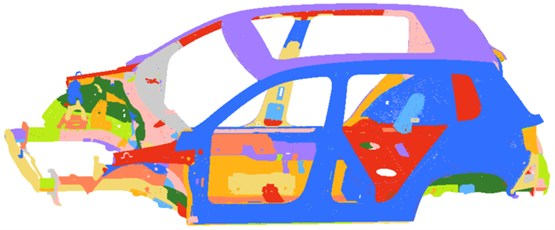Table 1Comparisons between experiment and simulation

 Order Simulation value / Hz Experiment value / Hz Mode Relative Error / % 1 30.8 31.31 Top dislocation –1.62 2 38.0 36.67 First-order torsion 3.62 3 40.0 38.41 First-order bending 4.14 4 42.9 44.29 Horizontal sway of front cabin –3.14

Fig. 2Modes of body in white at first 4 orders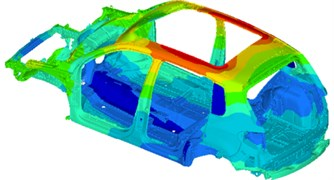a) 30.8 Hz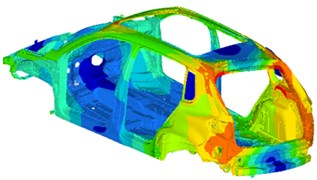b) 8.0 Hz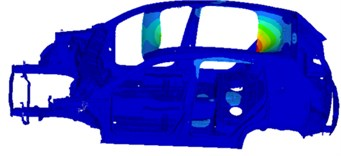c) 40.0 Hz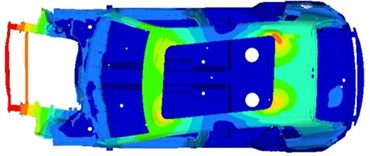d) 42.9Hz

### 3.2. The boundary element model

The boundary element model can be used to compute the sound field of the enclosed cavity and the opened cavity. The boundary element model required shell elements. During computing the sound field in the vehicle, it was generally processed as an enclosed cavity. When the fluid simulation was conducted in Virtual.Lab, behaviors such as reflection, diffraction and refraction of sounds were taken into account. Therefore, errors of the computational results mainly come from inaccuracy of material definitions, insufficient model and boundary conditions. In order to ensure that the largest size of an acoustic element was smaller than 1/6 of the shortest wavelength of the computational frequency, the element length was set to be 60 mm-70 mm. Acoustic meshes shall be basically consistent. The computational accuracy of a fluid model was controlled by most elements, so the size of elements should be uniform. The partial fine meshes cannot increase the computational accuracy. However, meshes can be divided appropriately in a more refined form at these places where the structure model was coupled with the fluid model.

In this paper, the boundary element model was built with the following steps: Firstly, the finite element meshes were enclosed; cavity meshes in the vehicle were extracted; Face command was then used to extract meshes on cavity boundaries; and the simplified seat meshes were also added into the model.

Fig. 3Boundary element model of the vehicle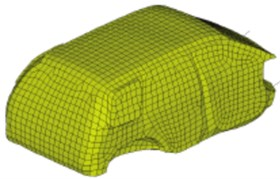Through the experiment, forces which were loaded by the engine onto 4 suspension points were shown in Fig. 4. The excitation force was applied at corresponding positions of the finite element model in Fig. 1. Then, the model was input into Virtual.Lab and coupled with the boundary element model in Fig. 3. Vibration characteristics of the structure were mapped into the boundary element model. In this way, the boundary element meshes can obtain all characteristics of the structural model and realize the vibro-acoustic coupling. 4 field points were set in the boundary element model and stood for driver, co-driver, left passenger and right passenger. Sound pressures at all passengers could be observed. Results were presented in Fig. 5. It was shown in Fig. 5 that sound pressure levels basically had the same tendencies at 4 field points, where 6 sound pressure peaks were presented, and the corresponding frequencies were 51 Hz, 110 Hz, 245 Hz, 262 Hz, 330 Hz and 382 Hz, respectively. The maximum value of sound pressure levels was more than 85 dB, but the minimum value was only 50 dB. Their difference approached 40 dB, and serious roar sound would be presented in the vehicle. Therefore, it was necessary to take some measures to reduce 6 peak noises.

In order to verify the effectiveness of the computational model, noises were tested in a semi-anechoic chamber, as shown in Fig. 6. The experimental environment had very large influences on the results. The semi-anechoic chamber as an acoustic lab was used to simulate a semi-free sound field. In the space, there were only direct sounds and primary reflection sounds. The experimental method was shown as follows: in the semi-anechoic chamber, the vehicle stably ran under an idling condition, while sound pressures of driver, co-driver, left passenger and right passenger were tested and recorded. During the experiment, sound pressure signals were collected by a piezoelectric microphone [12, 13]. The experimental system was LMS Test.Lab. Sampling frequency was 25600 Hz and the resolution ratio was 2 Hz. After completing collection, time-domain signals were processed by spectral analysis and compared with computational results. With sound pressures of the driver as an example, results were shown in Fig. 7.

It was shown in Fig. 7 that the consistency was good, but there were some errors between simulation and experiment mainly because that the boundary conditions of the computational model could hardly be kept consistent with actual situations.

Fig. 4Excitation forces of 4 points on the body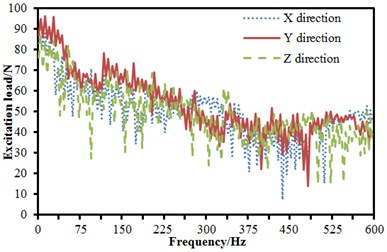a) Point 1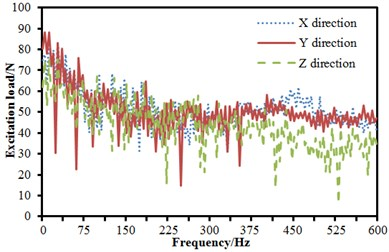b) Point 2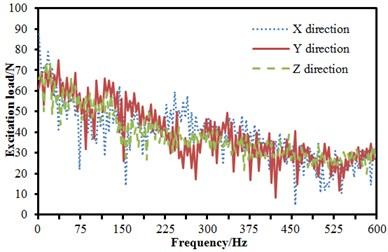c) Point 3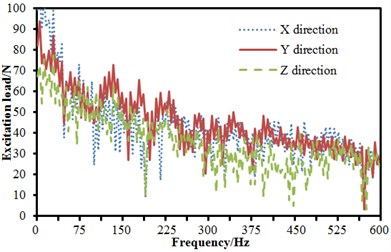d) Point 4

Fig. 5Sound pressure levels at all passengers in the vehicle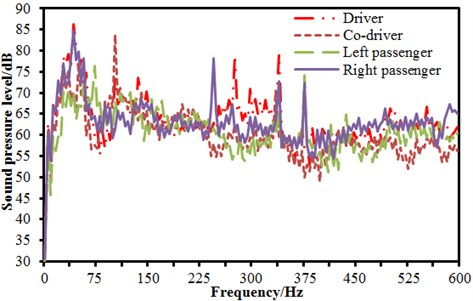Fig. 6The experimental noise in the vehicle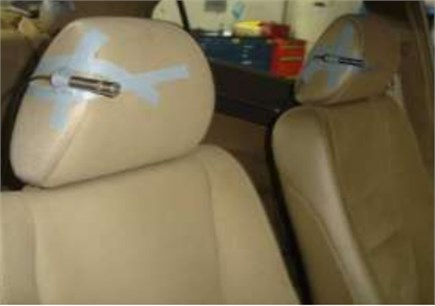Fig. 7Comparison of noises between experiment and simulation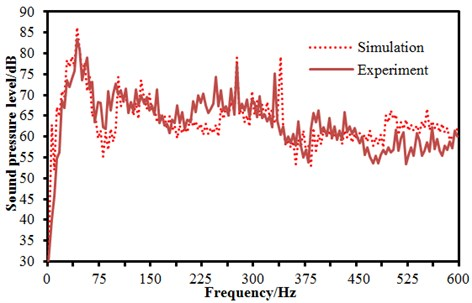Fig. 8Contribution color bar of panels in field points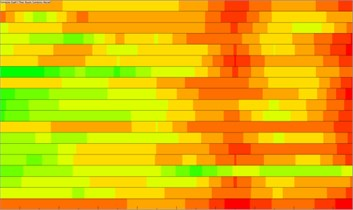a) Driver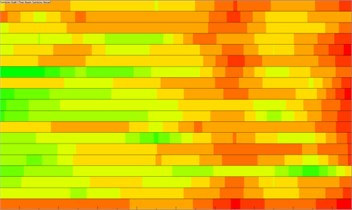b) Co-driverr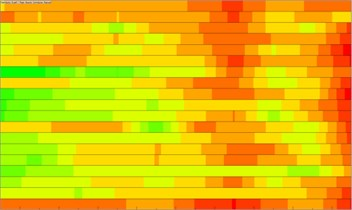c) Left passenger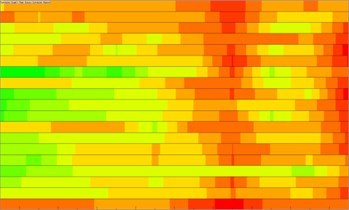d) Right passenger

Noises were always caused by vibrations of body panels. Therefore, in order to reduce noises in the vehicle, it was necessary to adopt some measures to reduce radiation noises of panels. ATV method mentioned in Section 2 can effectively recognize the panels which made large contributions to noises. Therefore, noises can be effectively improved by taking some measures to these panels. Firstly, acoustic cavity panels were grouped according to functions and characteristics of each panel, as shown in Table 2. Based on the vibro-acoustic coupling model, the panel contribution was conducted. Contributions made by panels at each field point were shown in Fig. 8. Contributions made by panels at each frequency point could be obtained through further normalization of these contributions, and results were presented in Fig. 9. It was shown in Fig. 9 that panel contributions at 6 peak frequency points were not completely consistent. As can be seen from this figure, it was also difficult to recognize the panels which made large contributions to peak noises. Therefore, it was necessary to adopt methods based on the information to recognize the panels which had large influences on noises of multi-characteristic frequency points.

Table 2ID of acoustic cavity panels

 Panel ID Panel Panel ID Panel 1 Firewall 10 Top panel 2 Front floor 11 Left front door 3 Middle floor 12 Right front door 4 Rear floor 13 Left rear door 5 Left rear wheel hood 14 Right rear door 6 Right rear wheel hood 15 Front wind shield 7 Back door inner panel 16 Left side 8 Rear wind shield 17 Right Side 9 Skylight glass 18 Dash panel

Fig. 9Panel contributions at 6 peak frequency points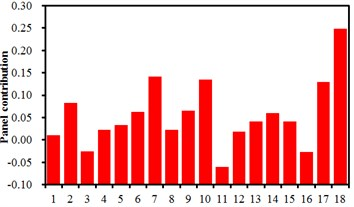a) 51 Hz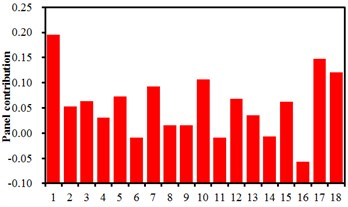b) 110Hz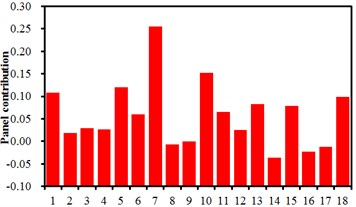a) 51Hz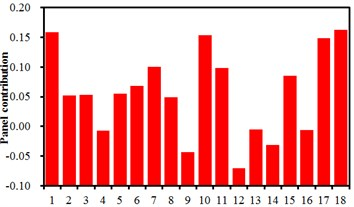b) 110Hz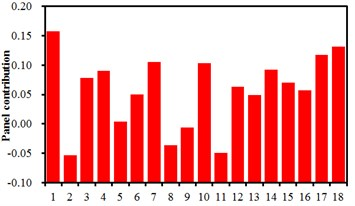e) 330Hz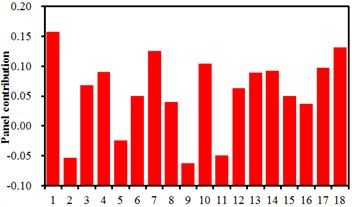f) 382Hz

## 4. Acoustic contributions of multi-characteristic frequencies

Based on the mentioned analysis, different acoustic contributions were made by body panels to field points under different characteristic frequencies. However, the importance of field points and characteristic frequencies in the comprehensive sound field in the vehicle can be relied on, and different weight can be given to every filed point and every response characteristic frequency. An expression of the acoustic contribution was proposed in the paper.

4
${\left({P}_{c}\right)}_{Total}={\sum }_{N=1}^{m}\left({\beta }_{N}{\sum }_{i=1}^{n}{\alpha }_{i}\bullet {\left({P}_{c}\right)}_{i,N}\right),$

wherein, ${\left({P}_{c}\right)}_{Total}$ was the total contribution of all panels to the comprehensive sound field under multi-characteristic frequencies; ${\left({P}_{c}\right)}_{i,N}$ was the acoustic contribution of all divided panels at a characteristic frequency $i$ of a field point $N$; $n$ was the number of characteristic frequencies related to the contribution analysis of panels; ${\alpha }_{i}$ was the weight coefficient of each characteristic frequency, which was used to distinguish different importance degrees of characteristic frequencies; and ${\beta }_{N}$ was the weight coefficient of the $N$th field point, which was determined by the importance of this field point in the sound field. This weight coefficient can be determined by the ratio of the sound pressure response at main characteristic frequency of the field point $N$ occupied in the total sound pressure response of all field points, and ${\beta }_{N}$ can be expressed as follows:

5
${\beta }_{N}=\frac{{\sum }_{i=1}^{n}{D}_{N,i}}{{\sum }_{i=1}^{n}{D}_{1,i}+{\sum }_{i=1}^{n}{D}_{2,i}+\dots +{\sum }_{i=1}^{n}{D}_{m,i}}=\frac{{\sum }_{i=1}^{n}{D}_{N,i}}{{\sum }_{N=1}^{m}{\sum }_{i=1}^{n}{D}_{N,i}},$

wherein, ${D}_{N,I}$ was the sound pressure response of the field point $N$ at the characteristic frequency $i$. The influence of panels on the acoustic characteristics of the sound field could be fully characterized by the comprehensive sound field contribution under multi characteristic frequencies in Eq. (4). Its magnitude was applied to represent this panel contribution to the comprehensive acoustic characteristics. Combined with the studied vehicle, the panel area was 18, $n$ = 6, $m$ = 4; the same degree of attention was paid to every characteristic frequency, ${\alpha }_{i}$ =1; in line with Eq. (5), the weights of all field points could be computed and obtained as Table 3. The comprehensive acoustic contributions of panels were ranked as Fig.10. It was shown in the figure that panels with ID of 1, 7, 10, 17 and 18 had large influences on 6 peak noises, and the corresponding panels were firewall, back door inner panel, top panel, right side and dash panel, respectively. Therefore, 6 peak noises can be effectively reduced through applying noise reduction measures to these panels, so that the environment can be improved.

Table 3Weight coefficient βN of each field point

 Field point Driver Co-driver Left passenger Right passenger ${\beta }_{N}$ 0.35 0.26 0.21 0.18

Fig. 10Panel contribution of the sound field under multi-featured frequencies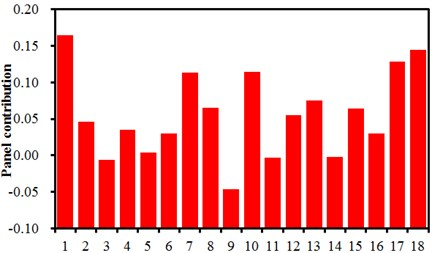## 5. Numerical optimization of noises based on the improved genetic algorithm

Through ATV analysis, panels which had serious influences on 6 peak noises in the vehicle have been recognized. Optimization design of these panels was conducted. As a result, their vibration characteristics can be reduced, and radiation noises can be improved.

### 5.1. Analysis of optimization problems

In the boundary element model, the interior parts were taken into account, and each panel was covered by a layer of damping structure. Therefore, the optimization mainly considered thicknesses of panels and damping materials to improve vibration effects. Stiffness of a panel mainly depended on the first order frequency. Therefore, during optimization design, it was necessary to ensure that the frequency of the optimized panel was more than that of the original structure. In addition, the mass of the optimized panel should not be higher than 50 % of mass of the original structure. Vibration velocities of 5 key panels needed to be optimized, so that it was a multi-objective optimization problem [14-17].

6
$\mathrm{min}f\left(x\right)=\mathrm{min}\left\{{\upsilon }_{1}\left(a\right),{\upsilon }_{2}\left(b\right),{\upsilon }_{3}\left(c\right),{\upsilon }_{4}\left(d\right),{\upsilon }_{5}\left(e\right)\right\},$
$a=\left\{{a}_{1},{a}_{2}\right\},b=\left\{{b}_{1},{b}_{2}\right\},c=\left\{{c}_{1},{c}_{2}\right\},d=\left\{{d}_{1},{d}_{2}\right\},e=\left\{{e}_{1},{e}_{2}\right\},$
${\omega }_{1}\ge 39.1,{\omega }_{2}\ge 26.3,{\omega }_{3}\ge 27.4,{\omega }_{4}\ge 36.1,{\omega }_{5}\ge 41.2,$
${m}_{1}\le 17.5,{m}_{2}\le 12.7,{m}_{3}\le 13.9,{m}_{4}\le 16.2,{m}_{5}\le 10.5,$
$0\le {a}_{i}\le 1,0\le {b}_{i}\le 1,0\le {c}_{i}\le 1,0\le {d}_{i}\le 1,0\le {e}_{i}\le 1,$
$i\in \left(1,2\right).$

### 5.2. Selection of optimization algorithm

The paper tried to use the genetic algorithm [18-21] to research this optimization problem. Its necessity was mainly presented as follows.

The genetic algorithm has been widely applied in the multi-objective optimization problem. It was a relatively mature optimization algorithm, and the operations were relatively convenient.

In addition, the commercial software ISIGHT was used in the optimization in the paper. Modules of genetic algorithm and simulated annealing algorithm were integrated in the software. Through an intermediate program, the simulated annealing algorithm can be integrated into the genetic algorithm. It was unnecessary to separately program a complex program. In this way, time was saved, the computational efficiency was increased, and cost was also reduced.

Fig. 11Flow diagram of the improved genetic algorithm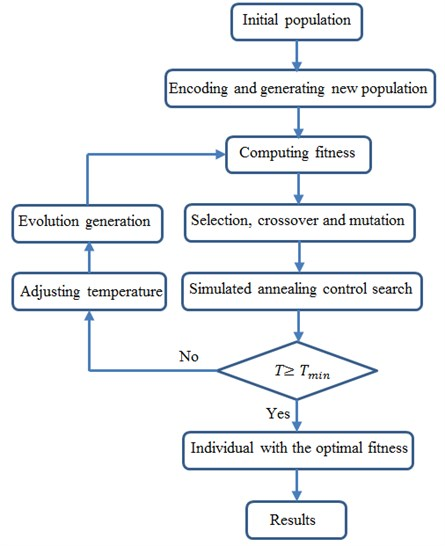During the optimization, the initial size of the population was set to 45, the crossover rate was 0.95, and the mutation rate was 0.05. As an important parameter in the optimization design process, the fitness reflected the convergence speed of the genetic algorithm. The fitness was changed with the evolutionary generation number. It can be obtained that the fitness value was gradually stabilized when the evolution generation was 75. However, the fitness value in the traditional genetic algorithm was generally stabilized and the result was converged after 240 generations. Therefore, it was presented that the improved genetic algorithm had obvious advantages in the multi-objective optimization.

### 5.3. Analysis of the optimized result

Based on the mentioned optimization, the thickness of each penal can be obtained. The optimized panels were applied in the vehicle to compute sound pressure levels at 4 field points.

A comprehensive indicator was introduced to express the differences before and after optimization because it was aimed at observing the improved effect of multiple field points:

7
${P}_{syn}\left(\omega \right)=\sqrt{{\sum }_{N=1}^{m}{P}_{N}{\left(\omega \right)}^{2}},$

wherein: ${P}_{syn}$ was the comprehensive noise indicator of multiple field points in the vehicle, and ${P}_{N}\left(\omega \right)$ was the noise spectrum of field point $N$.

Comparisons of the interior noise before and after optimization were shown in Fig. 12. As can be seen from the results, the noise has been reduced obviously. In addition, the total noises at 6 characteristic frequencies before and after optimization were listed in Table 4. SPLs of each field point were further presented in Fig. 13, and they were obviously improved. Therefore, the optimization method proposed in the paper was effective.

Fig. 12Comparisons of spectrum noises before and after optimization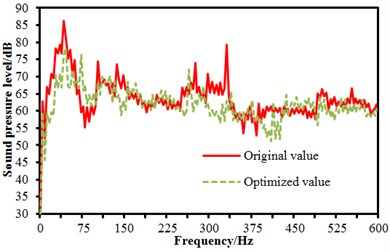a) Driver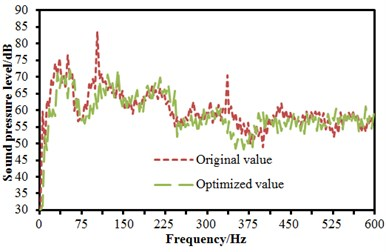b) Co-driver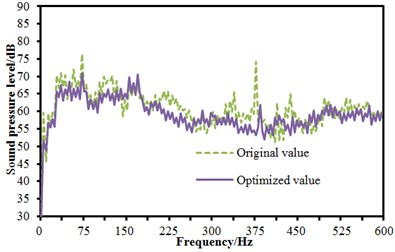c) Left passenger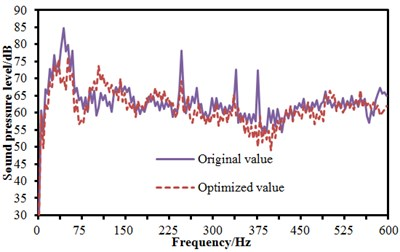d) Right passenger

Fig. 13Comparisons of average noises before and after optimization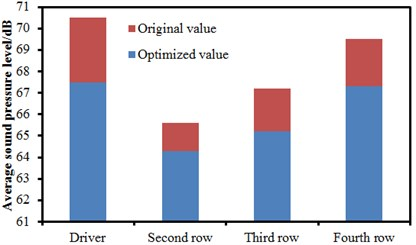Table 4Noises at 6 characteristic frequencies before and after optimization

 Frequency Before optimization After optimization Change 51 Hz 86.3 dB 78.5 dB –7.8 dB 110 Hz 84.8 dB 73.6 dB –11.2 dB 245 Hz 79.4 dB 72.3 dB –7.1 dB 262 Hz 78.4 dB 70.3 dB –8.1 dB 330 Hz 80.2 dB 68.5 dB –11.7 dB 382 Hz 75.1 dB 65.2 dB –9.9 dB

## 6. Conclusions

The finite element model of Body in White was built, and the corresponding modes were computed in this paper. These computational modes were then compared with experimental results. The small errors showed that the accuracy of the finite element model can satisfy the computational requirements. Based on the verified finite element model, acoustic cavities in the vehicle were extracted to build a boundary element model. Sound pressure levels at all passengers in the vehicle were then computed, compared and analyzed. Results indicated that the sound pressure curve had 6 peak noises. Using the characteristic frequency weight coefficient and field point weight coefficient, the body panels which made large acoustic contributions to the comprehensive sound field under multi-characteristic frequencies were determined.

Genetic Algorithm (GA) was a self-adaptive global search algorithm which was formed based on generation and evolution in a natural environment. This algorithm was outstanding in global search capability but insufficient in the local search capability. Simulated annealing (SA) was an algorithm featured with the outstanding local search ability and widely used in combinational optimization problems. As a result, they were combined to present their mutual advantages and use the global search capability of GA and local search capability of SA. The improved GA was used to optimize this problem in this paper.

Finally, the optimal combination of panel and damping material was determined. It was shown from the computational results of the optimized proposal that the interior noise was improved obviously. Therefore, the panel optimization based on the comprehensive sound field proposed in the paper was effective, which also provided a more complete and systematic optimization method for the vibration noise reduction of body panels.

#### Cited by

Vehicle Interior Noise Prediction Based on Elman Neural Network

24 November 2015
Accepted
16 June 2016
Published
30 June 2016
SUBJECTS
Acoustics, noise control and engineering applications
Keywords
panel contribution
acoustic transfer vector
improved genetic algorithm
multi-characteristic frequencies
Acknowledgements

This work was supported by Heilongjiang Provincial Natural Science Foundation (No. F201422).